Custom

# How to Calculate Correct Motor SizeNovember 21, 2019 by Seth Schaffer
Share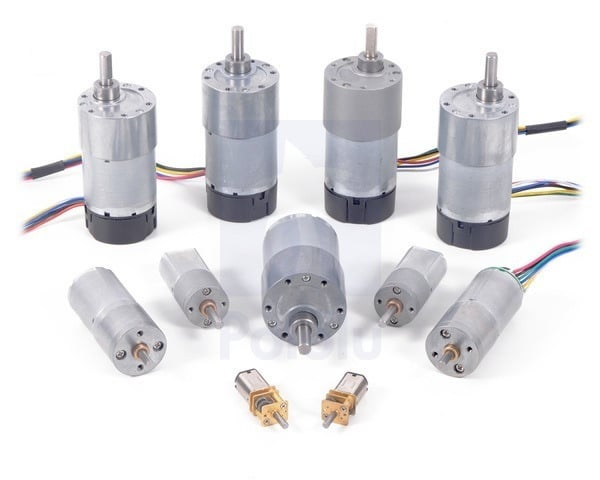Learn the math behind the decision-making process of robotic motor design with this in-depth article!

In the last article, I discussed how to size motors for two of the most common applications: drive systems and actuators. We talked about mechanical power and power curve. With that information in mind, let’s dive into the actual calculations and assumptions used for making motor design decisions with real-life examples.

For reference, there are a few resources that explain the calculations used here in detail. I am just going to explain the basics, ignoring mechanical inefficiencies.

## Drivetrain Calculations

For drive, you want your motor to have enough torque to spin the wheels. This is determined by the weight of the bot, how much of that is on a driven wheel, the wheel diameter, and the coefficient of friction between the floor and wheel. The wheel rpm is motor rpm/gear ratio, and wheel torque is motor torque * gear ratio. This means the force where the wheel touches the floor is the friction coefficient times torque divided by the wheel radius.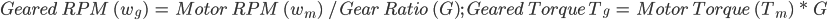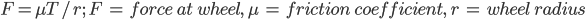From here, Newton’s Second Law tells you acceleration (F = ma).

Let’s say we have a 4-inch diameter wheel we want spinning at 600RPM with the CIM motor. What force can we generate from this with a coefficient of friction of 0.9 (roughly rubber on steel or wood)? We will assume we are just starting to move so rpm = 0 and torque = 2.41 N*m.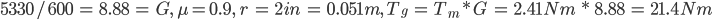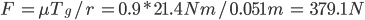If we have two driven wheels we can double this force and determine the acceleration from stall. This force also tells us the max pushing force our robot can exert. Let’s say our robot weighs 50 kg (110 lb) and we want to see how fast it accelerates with two wheels driven in this manner.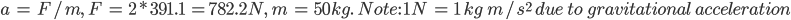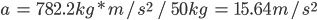This number may be realistic but it’s incredibly fast because it is only true the moment the motor starts turning. As soon as it picks up speed, the torque drops linearly according to that curve.

Eventually, the motor only delivers enough torque to move the robot at a constant speed, which is the top speed. If this weren’t true the robot would keep accelerating forever, which as we all know, can’t happen.

The speed of your bot is the wheel circumference2rtimes the rpm, with unit conversions where needed. For example, a 4-inch diameter wheel spinning at 600 rpm would be:

r = 2in, wg = 600rev/min * 1min/60sec = 10 rev/sec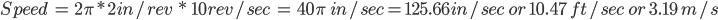While knowing these calculations is a good way to get a sense of the size of the motor needed and a ballpark for what stall torque is needed, using a ready-made calculator is far easier than working out these numbers for every motor you look at.

You can also take some shortcuts by assuming you want to operate a bit below max rpm for a given motor and ensure it has enough torque to accelerate your robot at a semi-reasonable pace at startup.

When designing your bot, you need to know the ballpark for how much it will weigh and what top speed you want, and if it needs to carry any extra weight lump that in as the robot weight. You will also likely know your wheel size from your design, meaning you can know what geared rpm you’re looking for.

For use in a robot drivetrain, you determine your gear ratio by choosing a target speed and gearing it to be slightly faster at the no-load RPM. So (in real life) with a load, you run close to the peak efficiency point.

### Drivetrain Resources

One great resource can be found at Ask Aaron. There, you can find a great article about optimizing the gearing for a robot drivetrain and a combat robot drivetrain calculator that makes it easy to determine what wheel diameter and gearing is required.

If you need your robot to work on slopes and not just flat ground, this Drive Motor Sizing Tool will help you out as well. However, its battery capacity calculator assumes that you have a coefficient of friction of one and that the motor is basically operating at full load 100% of the time so it vastly overestimates capacity.

## Robotic Actuator Calculations

Calculations for lifting masses on an extended arm are much more straightforward to do by hand.

The force/acceleration calculation from the previous section can be reused by making friction = 1 for positive engagement. All you need is the motor torque and length of your lever arm to determine what force can be lifted.

Then, take advantage of the fact that, with electric motors, speed and torque are inversely proportional. This means that you simply want to know if you are gearing your motor to a suitable torque for moving the load at your ideal speed. For example, if you want to move a mass of 1kg at the end of a 0.5m arm, then you only need 0.5kg*m of torque or 4.9Nm minimum (gravity applying a force of 9.8N/kg).

If you need the arm to move at a minimum speed of one rev/sec at that loading, the motor needs to be geared at 60 rpm or faster when generating this torque (‘or faster’ since torque increases as it slows down). For example, if you want to use a CIM motor to lift a 1kg mass at an extension of 0.5 meters: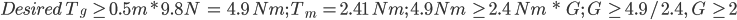Test Case: G = 3, T1=4.9/3 =1.63 Nm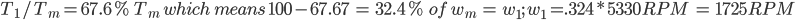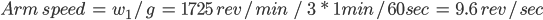With these results, we know with a gear ratio of 3:1 the CIM can easily supply the torque needed at over nine times the required minimum speed! We can use a higher ratio and still move plenty fast and raise the lifting capability as needed.

You need to also account for the weight of the arm itself which acts as a point mass at around half its length (or center of gravity). Keep in mind that if you are lifting a proportionally lighter load, the motor will be able to turn proportionally faster. If you design around a load of X, and actually lift a load of 80% of X, the motor will be able to spin about 1/.8 = 125% the speed and will draw about 80% as much current due to producing 80% as much torque. This won’t necessarily be perfectly accurate due to losses from friction and motor efficiency, but it will hold mostly true for loads that don’t drastically differ.

## Counterbalance in Actuators

One consideration for using motors as actuators is counterbalance. If you are always moving an offset mass that doesn’t change much, having a counterweight that counterbalances the torque from the load can drastically reduce the load on your joint.

Adding counterweight often requires a bulky mass, but could also be a spring or bungee cable which simply takes load off of the motor. This allows you to get away with a much weaker motor and reduce the torque needed to rotate your joints.

## Powering a Robot's Motor

At this point, I’ve covered most of the typical robotics uses for rotating motion and how to properly design the systems electrically to meet the basic mechanical demands of a project.

There are obviously a lot of other forms of motion, as well as other types of motors, such as steppers and servos, but these tend to be limited to 3D printers/CNC machines and smaller, low-power projects and there are plenty of other resources to turn to for those.

There is one more electrical subject that needs to be discussed in reference to these larger projects however: how to power them. Unlike simple LED displays, you won’t be able to use a glorified USB power bank for these 12V and higher systems. The same battery that works for a drone obviously won’t for a go-kart. In the next section of this series, I’ll be doing a deep dive into battery technology and how to find a suitable power source for your project.

Cover image courtesy of Pololu.

### AuthorSeth Schaffer

I am a professional Mechanical Engineer with a passion for combat robotics. I use CAD tools, CNC and 3D printing to create bots 3 to 30+ pounds.